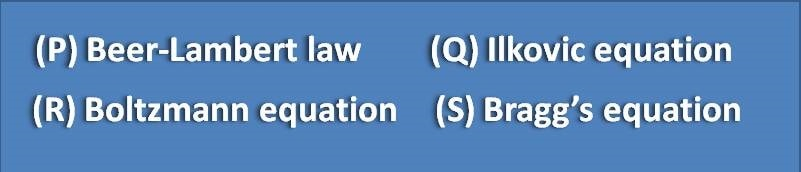1. Home
2. â€º
3. Test papers
4. â€º

# MCQ on polarography: Page-3

Which of the following are used to suppress the maxima in polarography(P) Starch(Q) Gelatin (R) Triton X-100(S) KCl

(A) Q,S

(B) P,R

(C) Q,R

(D) P,S

A sample contains two different ionic species at different concentrations. The two ions can be distinguished in polarography by

(A) Half-wave potentials

(B) Diffusion currents

(C) Polarography is confined to solutions containing single type of ions only

(D) By addition of one ion such that their concentrations are equal

Auxillary electrode is used in polarography to

(A) Dampen the residual current

(B) To dampen the current passing through reference electrode

(C) Dampen the migration current

(D) Dampen the convection current

The diffusion current in polarography is expressed by the equation(A) P

(B) Q

(C) R

(D) S

Capillary constant in the Illkovic equation is equal to

(A) m 1/2 t 1/6

(B) D 1/2 t 1/6

(C) m 2/3 t 1/6

(D) D 1/2 t 1/3